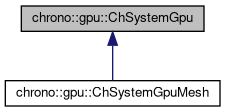chrono::gpu::ChSystemGpu Class Reference

## Description

Interface to a Chrono::Gpu system.

#include <ChSystemGpu.h>

Inheritance diagram for chrono::gpu::ChSystemGpu:[legend]

## Public Member Functions

ChSystemGpu (float sphere_rad, float density, float3 boxDims)
Construct system with given sphere radius, density, and big domain dimensions.

void SetGravitationalAcceleration (const ChVector< float > g)
Setr gravitational acceleration vector.

void SetParticlePositions (const std::vector< ChVector< float >> &points, const std::vector< ChVector< float >> &vels=std::vector< ChVector< float >>(), const std::vector< ChVector< float >> &ang_vels=std::vector< ChVector< float >>())
Set particle positions.

void SetBDFixed (bool fixed)
Set the big domain to be fixed or not. More...

void SetParticleFixed (const std::vector< bool > &fixed)
Set flags indicating whether or not a particle is fixed. More...

void SetOutputMode (CHGPU_OUTPUT_MODE mode)
Set the output mode of the simulation.

void SetOutputFlags (unsigned char flags)
Set output settings bit flags by bitwise ORing settings in CHGPU_OUTPUT_FLAGS.

void SetFixedStepSize (float size_UU)
Set timestep size.

void DisableMinLength ()
Ensure that the deformation-based length unit is used.

void SetTimeIntegrator (CHGPU_TIME_INTEGRATOR new_integrator)
Set the time integration scheme for the system.

void SetFrictionMode (CHGPU_FRICTION_MODE new_mode)
Set friction formulation. More...

void SetRollingMode (CHGPU_ROLLING_MODE new_mode)
Set rolling resistence formulation. More...

void SetStaticFrictionCoeff_SPH2SPH (float mu)
Set sphere-to-sphere static friction coefficient.

void SetStaticFrictionCoeff_SPH2WALL (float mu)
Set sphere-to-wall static friction coefficient.

void SetRollingCoeff_SPH2SPH (float mu)
Set sphere-to-sphere rolling friction coefficient – units and use vary by rolling friction mode.

void SetRollingCoeff_SPH2WALL (float mu)
Set sphere-to-wall rolling friction coefficient – units and use vary by rolling friction mode.

void SetSpinningCoeff_SPH2SPH (float mu)
Set sphere-to-sphere spinning friction coefficient – units and use vary by spinning friction mode.

void SetSpinningCoeff_SPH2WALL (float mu)
Set sphere-to-wall spinning friction coefficient – units and use vary by spinning friction mode.

void SetKn_SPH2SPH (double someValue)
Set sphere-to-sphere normal contact stiffness.

void SetKn_SPH2WALL (double someValue)
Set sphere-to-wall normal contact stiffness.

void SetGn_SPH2SPH (double someValue)
Set sphere-to-sphere normal damping coefficient.

void SetGn_SPH2WALL (double someValue)
Set sphere-to-wall normal damping coefficient.

void SetKt_SPH2SPH (double someValue)
Set sphere-to-sphere tangential contact stiffness.

void SetGt_SPH2SPH (double someValue)
Set sphere-to-sphere tangential damping coefficient.

void SetKt_SPH2WALL (double someValue)
Set sphere-to-wall tangential contact stiffness.

void SetGt_SPH2WALL (double someValue)
Set sphere-to-wall tangential damping coefficient.

void SetCohesionRatio (float someValue)
Set the ratio of cohesion to gravity for monodisperse spheres. Assumes a constant cohesion model.

Set the ratio of adhesion to gravity for sphere to wall. Assumes a constant cohesion model.

void SetMaxSafeVelocity_SU (float max_vel)
Safety check velocity to ensure the simulation is still stable.

void SetPsiFactors (unsigned int psi_T, unsigned int psi_L, float psi_R=1.f)
Set tuning psi factors for tuning the non-dimensionalization.

void SetRecordingContactInfo (bool record)
Enable/disable recording of contact info.

void SetVerbosity (CHGPU_VERBOSITY level)
Set simualtion verbosity level.

size_t CreateBCSphere (const ChVector< float > &center, float radius, bool outward_normal, bool track_forces)
Create an axis-aligned sphere boundary condition.

size_t CreateBCConeZ (const ChVector< float > &tip, float slope, float hmax, float hmin, bool outward_normal, bool track_forces)
Create a Z-axis aligned cone boundary condition.

size_t CreateBCPlane (const ChVector< float > &pos, const ChVector< float > &normal, bool track_forces)
Create a plane boundary condition.

size_t CreateBCCylinderZ (const ChVector< float > &center, float radius, bool outward_normal, bool track_forces)
Create a Z-axis aligned cylinder boundary condition.

bool DisableBCbyID (size_t BC_id)
Disable a boundary condition by its ID, returns false if the BC does not exist.

bool EnableBCbyID (size_t BC_id)
Enable a boundary condition by its ID, returns false if the BC does not exist.

bool SetBCOffsetFunction (size_t BC_id, const GranPositionFunction &offset_function)
Enable a boundary condition by its ID, returns false if the BC does not exist.

void setBDWallsMotionFunction (const GranPositionFunction &pos_fn)
Prescribe the motion of the big domain, allows wavetank-style simulations.

float GetSimTime () const
Return current simulation time.

size_t GetNumParticles () const
Return the total number of particles in the system.

Return the radius of a spherical particle.

double GetMaxParticleZ () const
Return the maximum Z position over all particles.

ChVector< float > GetParticlePosition (int nSphere) const
Return particle position.

ChVector< float > GetParticleAngVelocity (int nSphere) const
Return particle angular velocity.

ChVector< float > GetParticleVelocity (int nSphere) const
Return particle linear velocity.

ChVector< float > GetBCPlanePosition (size_t plane_id) const
Return position of BC plane.

bool GetBCReactionForces (size_t BC_id, ChVector< float > &force) const
Get the reaction forces on a boundary by ID, returns false if the forces are invalid (bad BC ID)

int GetNumContacts () const
Return number of particle-particle contacts.

unsigned int GetNumSDs () const
Return number of subdomains in the big domain.

virtual void Initialize ()
Initialize simulation so that it can be advanced. More...

Advance simulation by duration in user units, return actual duration elapsed. More...

void WriteFile (std::string ofile) const
Write particle positions according to the system output mode.

void WriteContactInfoFile (std::string ofile) const
Write contact info file.

size_t EstimateMemUsage () const
Roughly estimate of the total amount of memory used by the system.

## Protected Member Functions

ChSystemGpu ()
Protected default constructor. Derived class must create m_sys.

## Protected Attributes

ChSystemGpu_impl * m_sys
underlying system implementation

## Member Function Documentation

 double chrono::gpu::ChSystemGpu::AdvanceSimulation ( float duration )
virtual

Advance simulation by duration in user units, return actual duration elapsed.

Requires Initialize() to have been called.

Reimplemented in chrono::gpu::ChSystemGpuMesh.

## ◆ Initialize()

 void chrono::gpu::ChSystemGpu::Initialize ( )
virtual

Initialize simulation so that it can be advanced.

Must be called before AdvanceSimulation and after simulation parameters are set.

Reimplemented in chrono::gpu::ChSystemGpuMesh.

## ◆ SetBDFixed()

 void chrono::gpu::ChSystemGpu::SetBDFixed ( bool fixed )

Set the big domain to be fixed or not.

If fixed, it will ignore any given position functions.

## ◆ SetFrictionMode()

 void chrono::gpu::ChSystemGpu::SetFrictionMode ( CHGPU_FRICTION_MODE new_mode )

Set friction formulation.

The frictionless setting uses a streamlined solver and avoids storing any physics information associated with friction.

## ◆ SetParticleFixed()

 void chrono::gpu::ChSystemGpu::SetParticleFixed ( const std::vector< bool > & fixed )

Set flags indicating whether or not a particle is fixed.

MUST be called only once and MUST be called before Initialize.

## ◆ SetRollingMode()

 void chrono::gpu::ChSystemGpu::SetRollingMode ( CHGPU_ROLLING_MODE new_mode )

Set rolling resistence formulation.

NOTE: This requires friction to be active, otherwise this setting will be ignored.

The documentation for this class was generated from the following files:
• /builds/uwsbel/chrono/src/chrono_gpu/physics/ChSystemGpu.h
• /builds/uwsbel/chrono/src/chrono_gpu/physics/ChSystemGpu.cpp